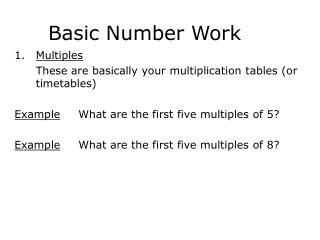DownloadDownload PresentationBasic Number Work

Basic Number Work

Télécharger la présentationBasic Number Work

- - - - - - - - - - - - - - - - - - - - - - - - - - - E N D - - - - - - - - - - - - - - - - - - - - - - - - - - -
Presentation Transcript

1. Basic Number Work Multiples These are basically your multiplication tables (or timetables) Example What are the first five multiples of 5? Example What are the first five multiples of 8?

2. 2. Lowest Common Multiple Example What is the lowest common multiple of 6 and 8? Example What is the lowest common multiple of 7 and 12?

3. 3. Factors A factor is any number that will divide exactly into a number given. Example What are the factors of 12? Example What are the factors of each of the following (a) 12 (b) 16 (c) 28

4. 4. Prime numbers A prime number is a number that can only be divided exactly into itself of one. That is any number which has only two factors is a prime number. The first six prime numbers are 2, 3, 5, 7, 11, 13

5. 5. Prime factors Example find the prime factors of 12 Example Find the prime factors for each of the following (a) 40 (b) 100 (c) 220 (d) 136 (e) 450

6. 6. Highest Common Factor Example What is the highest common factor of 16 and 24? Example What is the highest common factor of 48, 72 and 288?